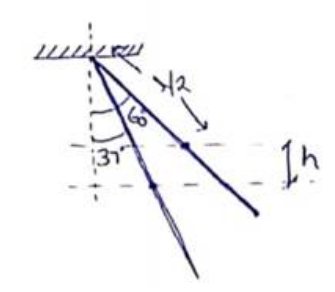Deepak Scored 45->99%ile with Bounce Back Crack Course. You can do it too!

# Solve the following :

Question:

A uniform rod pivoted at its upper end hangs vertically. It is displaced through an angle of $60^{\circ}$ and then released. Find the magnitude of the force acting on a particle of mass $\mathrm{dm}$ at the tip of the rod when the rod makes an angle of $37^{\circ}$ with the vertical.Solution:

By energy conservation

$\frac{1}{2} I \omega^{2}=m g h$

$\frac{1}{2}\left(\frac{m L^{2}}{3}\right) \omega^{2}=m g \frac{l}{2}\left(\cos 37^{\circ}-\cos 60^{\circ}\right)$

$\omega^{2}=\frac{9 g}{10 I}$

So, centrifugal force

$F_{C}=(d m) l \omega^{2}$

$F_{C}=0.9 g(d m)$

Let angular acceleration of the rod be $\alpha$ when it makes an angle of $37^{\circ}$ with the vertical. $\mathrm{T}=\mid \alpha$

$\operatorname{mg}\left(\frac{l}{2} \sin 37^{2}\right)=\left(\frac{m L^{2}}{3} \alpha\right)$

$\alpha=0.9\left(\frac{g}{q}\right)$.

So, tangential force

$F_{t}=(d m)\left(a_{t}\right)$

$=(d m)(l \alpha)$

$F_{t}=0.9 g(d m)$

So,

$F_{N}=\sqrt{F_{t}^{2}+F_{C}^{2}}$

$F_{N}=0.9 \sqrt{2 g}(d m)$